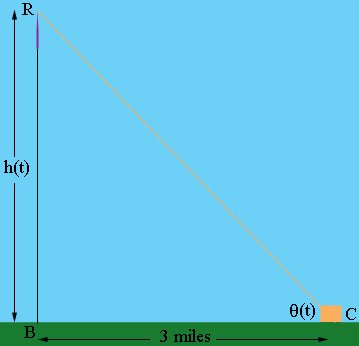SEARCH HOMEMath Central Quandaries & QueriesQuestion from MJ, a student: A rocket that is rising vertically is being tracked by a ground level camera located 3 mi from the point of blast off when the rocket is 2 mi high its speed is 400mph At what rate is the (acute) angle between the horizontal and the camera's line of sight changingHi again MJ,

This problem is quite similar to the previous one you sent. In this problem I would draw a diagram.$R$ is the rocket at time $t,$ $B$ is the blastoff point and $C$ is the camera. $h(t)$ is the height of the rocket in miles at time $t.$ The angle between the horizontal and the line of sight of the camera I called $\theta(t).$

You are asked to find the rate of change of $\theta(t)$ at the time when $h(t) = 2$ miles and $h^\prime(t)$ is 400 miles per hour. To do this you need to find a relationship between $h(t)$ and $\theta(t).$ What trig function relates $h(t), \theta(t)$ and the side $BC$?

PennyMath Central is supported by the University of Regina and The Pacific Institute for the Mathematical Sciences.